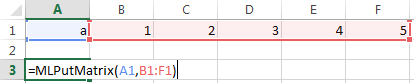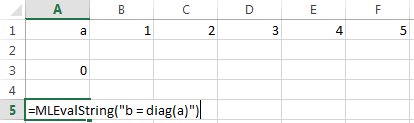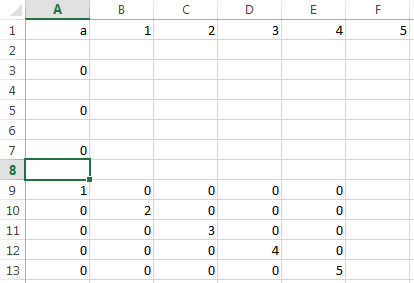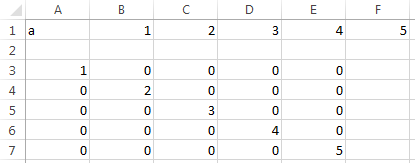MLEvalString

Evaluate MATLAB command in MATLAB

Description

example

= MLEvalString(command) specifies the MATLAB® command for evaluation in the MATLAB workspace. Use this syntax when working in a worksheet cell.

example

MLEvalString command works in a VBA macro.

example

err = MLEvalString(command) returns the execution status when executing MLEvalString in a VBA macro.

Examples

collapse all

Enter the variable a into cell A1. Enter the numbers 1 through 5 into the range of cells from B1 through F1.

Assign the range of cells to variable a in MATLAB using MLPutMatrix. Enter this text in cell A3.

=MLPutMatrix(A1,B1:F1)Use diag to create a matrix b, containing a diagonal using the five numbers in variable a. Enter this text in cell A5.

=MLEvalString("b = diag(a);")Retrieve matrix b from MATLAB into Excel® cell A9. Enter this text in cell A7.

=MLGetMatrix("b","A9")

The matrix with the diagonal appears in cells A9 through E13.Enter the variable a into cell A1. Enter the numbers 1 through 5 into the range of cells from B1 through F1.

Click the Developer tab in Microsoft® Excel, and then click . The Visual Basic® Editor window opens.

Insert a new module and enter this VBA code into the Code section of the window. This sample code assumes a macro named Diagonal. For details on working with modules, see Excel Help.

Sub Diagonal()

MLPutMatrix "a", Range("B1:F1")
MLEvalString "b = diag(a);"
MLGetMatrix "b", "A3"
MatlabRequest

End Sub

Run the macro by clicking . The diagonal matrix appears in cells A3 through E7. For details on running macros, see Excel Help.Enter the variable a into cell A1. Enter the numbers 1 through 5 into the range of cells from B1 through F1.

Click the Developer tab in Microsoft Excel, and then click . The Visual Basic Editor window opens.

Insert a new module and enter this invalid VBA code into the Code section of the window. This sample code assumes a macro named Diagonal. For details on working with modules, see Excel Help.

Sub Diagonal()

Dim err As Variant

MLPutMatrix "a", Range("B1:F1")
err = MLEvalString("b = diag(2a);") 'Invalid code

If err <> 0 Then
MsgBox err
End If

MLGetMatrix "b", "A3"
MatlabRequest

End Sub

Run the macro by clicking in the VBA toolbar. For details on running macros, see Excel Help.

This Spreadsheet Link™ error displays: #COMMAND!. To display MATLAB errors, see MLShowMatlabErrors.

Input Arguments

collapse all

MATLAB command to evaluate, specified as a string. Enclose the string in double quotes. Or, enter the string in a cell without quotes and enter the corresponding cell reference without quotes as the input argument.

Example: "sum"

Example: A1

Output Arguments

collapse all

Execution status, returned as a string or number. If MLEvalString fails, then err is a string containing an error code or error message. Otherwise, the command executes successfully and err is 0.

By default when MLEvalString fails, err contains a standard Spreadsheet Link error, such as #COMMAND. To return MATLAB errors, execute MLShowMatlabErrors.

Tips

• The specified action alters only the MATLAB workspace and has no effect on the Microsoft Excel workspace.

• To work with VBA code in Excel with Spreadsheet Link, you must enable Spreadsheet Link as a reference in the Microsoft Visual Basic Editor. For details, see Installation.

Introduced before R2006a